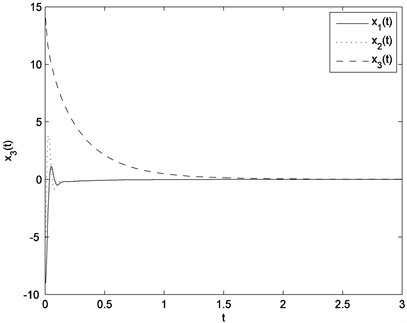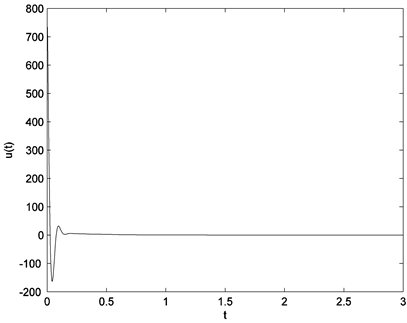﻿ 基于分数阶Takagi-Sugeno模糊模型的分数阶Chen混沌系统的控制

# 基于分数阶Takagi-Sugeno模糊模型的分数阶Chen混沌系统的控制Stability of Fractional Order Chen Chaos Based on Takagi-Sugeno Fuzzy Model

Abstract: The control problem of fractional order Chen chaos system based on fractional order Tak-agi-Sugeno fuzzy model is studied. The Takagi-Sugeno fuzzy model for fractional order Chen chaos systems is given, and a parallel distributed compensate fuzzy controller is designed to asymptotically stabilize the model. Then effectiveness of the approach is tested on fractional order Chen chaos system with α=0.95 .

1. 引言

2. 分数阶Takagi-Sugeno模糊系统

2.1. Caputo型分数阶导数

$\begin{array}{l}{}_{a}^{C}{D}_{t}^{\alpha }f\left(t\right)=\frac{1}{\Gamma \left(r-\alpha \right)}{\int }_{a}^{t}\frac{{f}^{\left(r\right)}\left(\tau \right)}{{\left(t-\tau \right)}^{\alpha +1-r}}\text{d}\tau ,\text{\hspace{0.17em}}\left(r-1\le \alpha (1)

2.2. 分数阶Takagi-Sugeno模糊系统

Takagi-Sugeno模糊模型自产生之日起就是处理非线性系统问题的有效方法之一。混沌系统是一类典型的非线性系统，本文利用分数阶Takagi-Sugeno模糊模型来研究非线性分数阶Chen混沌系统的控制问题。首先我们介绍分数阶Takagi-Sugeno模糊系统。

Rule i: If ${z}_{1}\left(t\right)$ is ${M}_{i1}$ and … ${z}_{p}\left(t\right)$ is ${M}_{ip}$ ;

Then $\left\{\begin{array}{l}{D}^{\alpha }x\left(t\right)={A}_{i}x\left(t\right)+{B}_{i}u\left(t\right)\hfill \\ x\left(0\right)={x}_{0}\hfill \end{array}$

$\left\{\begin{array}{l}{D}^{\alpha }x\left(t\right)=\underset{i=1}{\overset{r}{\sum }}{h}_{i}\left(z\left(t\right)\right)\left({A}_{i}x\left(t\right)+{B}_{i}u\left(t\right)\right)\hfill \\ x\left(0\right)={x}_{0}\hfill \end{array}$ (2)

$\left\{\begin{array}{l}\underset{i=1}{\overset{r}{\sum }}{w}_{i}\left(z\left(t\right)\right)>0\hfill \\ {w}_{i}\left(z\left(t\right)\right)\ge 0\hfill \end{array}$

$\left\{\begin{array}{l}\underset{i=1}{\overset{r}{\sum }}{h}_{i}\left(z\left(t\right)\right)=1\hfill \\ {h}_{i}\left(z\left(t\right)\right)\ge 0\hfill \end{array}$

${A}_{i}Q-{B}_{i}{M}_{i}+{\left({A}_{i}Q-{B}_{i}{M}_{i}\right)}^{\text{T}}<0,\text{\hspace{0.17em}}\text{\hspace{0.17em}}i=1,2,\cdots ,r$, (3)

${A}_{i}Q-{B}_{i}{M}_{j}+{A}_{j}Q-{B}_{j}{M}_{i}+{\left({A}_{i}Q-{B}_{i}{M}_{j}+{A}_{j}Q-{B}_{j}{M}_{i}\right)}^{\text{T}}<0,\text{\hspace{0.17em}}\text{\hspace{0.17em}}1\le i, (4)

3. 分数阶Chen混沌系统

3.1. 分数阶Chen混沌系统

$\left\{\begin{array}{l}{D}^{\alpha }{x}_{1}\left(t\right)=a\left({x}_{2}\left(t\right)-{x}_{1}\left(t\right)\right),\hfill \\ {D}^{\alpha }{x}_{2}\left(t\right)=\left(c-a\right){x}_{1}\left(t\right)-{x}_{1}\left(t\right){x}_{3}\left(t\right)+c{x}_{2}\left(t\right),\hfill \\ {D}^{\alpha }{x}_{3}\left(t\right)=-b{x}_{3}\left(t\right)+{x}_{1}\left(t\right){x}_{2}\left(t\right).\hfill \end{array}$ (5)Figure 1. Chaotic behaviors of the fractional order Chen system

3.2. 分数阶Chen混沌系统的Takagi-Sugeno模糊模型

Rule 1: If ${x}_{1}\left(t\right)$ is ${F}_{1}\left({x}_{1}\left(t\right)\right)$, then ${D}^{\alpha }x\left(t\right)={A}_{1}x\left(t\right)$ ;

Rule 2: If ${x}_{1}\left(t\right)$ is ${F}_{2}\left({x}_{1}\left(t\right)\right)$, then ${D}^{\alpha }x\left(t\right)={A}_{2}x\left(t\right)$.

${A}_{1}=\left[\begin{array}{ccc}-a& a& 0\\ c-a& c& -d\\ 0& d& -b\end{array}\right]$${A}_{2}=\left[\begin{array}{ccc}-a& a& 0\\ c-a& c& d\\ 0& -d& -b\end{array}\right]$

${F}_{1}\left({x}_{1}\left(t\right)\right)=\left(1/2\right)\left(1-{x}_{1}\left(t\right)/d\right)$

${F}_{2}\left({x}_{1}\left(t\right)\right)=\left(1/2\right)\left(1+{x}_{1}\left(t\right)/d\right)$

${D}^{\alpha }x\left(t\right)=\underset{i=1}{\overset{2}{\sum }}{F}_{i}\left(z\left(t\right)\right){A}_{i}x\left(t\right)$ (6)

$\left\{\begin{array}{l}\underset{i=1}{\overset{2}{\sum }}{F}_{i}\left({x}_{1}\left(t\right)\right)=1\hfill \\ {F}_{i}\left({x}_{1}\left(t\right)\right)\ge 0\hfill \end{array}$

3.3. 基于分数阶Takagi-Sugeno模糊系统的分数阶Chen混沌系统的控制

$\left\{\begin{array}{l}{D}^{\alpha }{x}_{1}\left(t\right)=a\left({x}_{2}\left(t\right)-{x}_{1}\left(t\right)\right),\hfill \\ {D}^{\alpha }{x}_{2}\left(t\right)=\left(c-a\right){x}_{1}\left(t\right)-{x}_{1}\left(t\right){x}_{3}\left(t\right)+c{x}_{2}\left(t\right)+u\left(t\right),\hfill \\ {D}^{\alpha }{x}_{3}\left(t\right)=-b{x}_{3}\left(t\right)+{x}_{1}\left(t\right){x}_{2}\left(t\right).\hfill \end{array}$ (7)

Rule 1: If ${x}_{1}\left(t\right)$ is ${F}_{1}\left({x}_{1}\left(t\right)\right)$, then ${D}^{\alpha }x\left(t\right)={A}_{1}x\left(t\right)+{B}_{1}u\left(t\right)$ ;

Rule 2: If ${x}_{1}\left(t\right)$ is ${F}_{2}\left({x}_{1}\left(t\right)\right)$, then ${D}^{\alpha }x\left(t\right)={A}_{2}x\left(t\right)+{B}_{2}u\left(t\right)$.

Rule 1: If ${x}_{1}\left(t\right)$ is ${F}_{1}\left({x}_{1}\left(t\right)\right)$, then $u\left(t\right)=-{K}_{1}x\left(t\right)$ ;

Rule 2: If ${x}_{1}\left(t\right)$ is ${F}_{2}\left({x}_{1}\left(t\right)\right)$, then $u\left(t\right)=-{K}_{2}x\left(t\right)$.

$u\left(t\right)=-\underset{i=1}{\overset{2}{\sum }}{F}_{i}\left({x}_{1}\left(t\right)\right){K}_{i}x\left(t\right),\text{\hspace{0.17em}}\text{\hspace{0.17em}}i=1,2$ (8)

${D}^{\alpha }x\left(t\right)=\underset{i=1}{\overset{r}{\sum }}\underset{j=1}{\overset{r}{\sum }}{h}_{i}\left(z\left(t\right)\right)\left({A}_{i}-{B}_{i}{K}_{j}\right)x\left(t\right)$ (9)

${A}_{1}Q-{B}_{1}{M}_{1}+{\left({A}_{1}Q-{B}_{1}{M}_{1}\right)}^{\text{T}}<0$

${A}_{2}Q-{B}_{2}{M}_{2}+{\left({A}_{2}Q-{B}_{2}{M}_{2}\right)}^{\text{T}}<0$

${A}_{1}Q-{B}_{1}{M}_{2}+{A}_{2}Q-{B}_{2}{M}_{1}+{\left({A}_{1}Q-{B}_{1}{M}_{2}+{A}_{2}Q-{B}_{2}{M}_{1}\right)}^{\text{T}}<0$ (10)

$Q=\left[\begin{array}{ccc}0.\text{1782}& 0.0\text{213}& -0.00\text{49}\\ 0.0\text{213}& 0.\text{4162}& 0.000\text{4}\\ -0.00\text{49}& 0.000\text{4}& 0.\text{5926}\end{array}\right]$

${M}_{1}=\left[\begin{array}{ccc}\text{12}.\text{8975}& \text{16}.\text{4537}& -\text{2}.\text{9931}\end{array}\right]$

${M}_{2}=\left[\begin{array}{ccc}\text{13}.0\text{224}& \text{16}.\text{2451}& \text{5}.\text{2164}\end{array}\right]$

${K}_{1}=\left[\begin{array}{ccc}\text{67}.\text{9598}& \text{36}.0\text{6}0\text{1}& -\text{4}.\text{511}0\end{array}\right]$

${K}_{2}=\left[\begin{array}{ccc}\text{69}.\text{1121}& \text{35}.\text{4868}& \text{9}.\text{352}0\end{array}\right]$

$u\left(t\right)=-\underset{i=1}{\overset{2}{\sum }}{F}_{i}\left({x}_{1}\left(t\right)\right){K}_{i}x\left(t\right),\text{\hspace{0.17em}}\text{\hspace{0.17em}}i=1,2$Figure 2. Control results of the fractional order Chen systemFigure 3. Control curve of the fractional order Chen system

4. 结论

 Lorenz, E.N. (1963) Deterministic Nonperiodic Flow. Journal of the Atmospheric Sciences, 20, 130-141.
https://doi.org/10.1175/1520-0469(1963)020<0130:DNF>2.0.CO;2

 Chen, G. and Ueta, T. (1999) Yet Another Chaotic Attractor. International Journal of Bifurcation and Chaos, 9, 1465-1466.
https://doi.org/10.1142/S0218127499001024

 Ueta, T. and Chen, G. (2000) Bifurcation Analysis of Chen’s Equation. International Journal of Bifurcation and Chaos, 10, 1917-1931.
https://doi.org/10.1142/S0218127400001183

 Sprott, J.C. (2015) New Chaotic Regimes in the Lorenz and Chen Systems. International Journal of Bifurcation and Chaos, 25, Article ID: 1550033.
https://doi.org/10.1142/S0218127415500339

 Zhang, F., Liao, X., Mu, C., et al. (2017) On Global Boundedness of the Chen System. Discrete & Continuous Dynamical Systems-B, 22, 1673.
https://doi.org/10.3934/dcdsb.2017080

 Algaba, A., Domínguez-Moreno, M.C., Merino, M., et al. (2015) Study of the Hopf Bifurcation in the Lorenz, Chen and Lü Systems. Nonlinear Dynamics, 79, 885-902.
https://doi.org/10.1007/s11071-014-1709-2

 Leonov, G.A. and Kuznetsov, N.V. (2015) On Differences and Similarities in the Analysis of Lorenz, Chen, and Lu Systems. Applied Mathematics and Computation, 256, 334-343.
https://doi.org/10.1016/j.amc.2014.12.132

 El-Sayed, A.M.A., Nour, H.M., Elsaid, A., et al. (2016) Dynamical Behaviors, Circuit Realization, Chaos Control, and Synchronization of a New Fractional Order Hyperchaotic System. Applied Mathematical Modelling, 40, 3516-3534.
https://doi.org/10.1016/j.apm.2015.10.010

 Odibat, Z., Corson, N., Aziz-Alaoui, M.A., et al. (2017) Chaos in Fractional Order Cubic Chua System and Synchronization. International Journal of Bifurcation and Chaos, 27, Article ID: 1750161.
https://doi.org/10.1142/S0218127417501619

 Khamsuwan, P., Sangpet, T. and Kuntanapreeda, S. (2018) Chaos Synchronization of Fractional-Order Chaotic Systems with Input Saturation. Journal of Computational and Nonlinear Dynamics, 13, Article ID: 090903.
https://doi.org/10.1115/1.4039681

 Čermák, J. and Nechvátal, L. (2019) Stability and Chaos in the Frac-tional Chen System. Chaos, Solitons & Fractals, 125, 24-33.
https://doi.org/10.1016/j.chaos.2019.05.007

 Takagi, T. and Sugeno, M. (1985) Fuzzy Identification of Systems and Its Applications to Modeling and Control. IEEE Transactions on Systems, Man, and Cybernetics, SMC-15, 116-132.
https://doi.org/10.1109/TSMC.1985.6313399

 Tanaka, K. and Sugeno, M. (1992) Stability Analysis and Design of Fuzzy Control Systems. Fuzzy Sets and Systems, 45, 135-156.
https://doi.org/10.1016/0165-0114(92)90113-I

 Asemani, M.H. and Vatankhah, R. (2017) NON-PDC Observer-Based T-S Fuzzy Tracking Controller Design and Its Application in CHAOS Control. Asian Journal of Control, 19, 969-982.
https://doi.org/10.1002/asjc.1451

 Yang, C.H., Wu, C.L., Chen, Y.J., et al. (2015) Reduced Fuzzy Controllers for Lorenz-Stenflo System Control and Synchronization. International Journal of Fuzzy Systems, 17, 158-169.
https://doi.org/10.1007/s40815-015-0032-5

 Chadli, M. and Zelinka, I. (2014) Chaos Synchronization of Unknown Inputs Takagi-Sugeno Fuzzy: Application to Secure Communications. Com-puters & Mathematics with Applications, 68, 2142-2147.
https://doi.org/10.1016/j.camwa.2013.01.013

 Podlubny, I. (1999) Fractional Differential Equations. Academic Press, San Diego, CA.

 Li, Y. and Li, J. (2014) Stability Analysis of Fractional Order Systems Based on T-S Fuzzy Model with the Fractional Order . Nonlinear Dynamics, 78, 2909-2919.
https://doi.org/10.1007/s11071-014-1635-3

Top